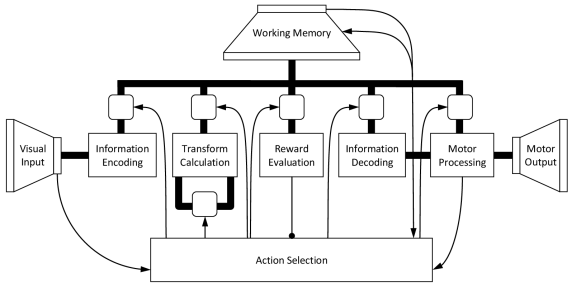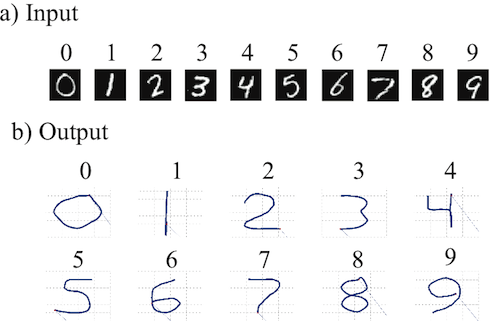# Introduction to the Semantic Pointer Architecture¶

Briefly, the Semantic Pointer hypothesis states:

Higher-level cognitive functions in biological systems are made possible by Semantic Pointers. Semantic Pointers are neural representations that carry partial semantic content and are composable into the representational structures necessary to support complex cognition.

The term ‘Semantic Pointer’ was chosen because the representations in the architecture are like ‘pointers’ in computer science (insofar as they can be ‘dereferenced’ to access large amounts of information which they do not directly carry). However, they are ‘semantic’ (unlike pointers in computer science) because these representations capture relations in a semantic vector space in virtue of their distances to one another, as typically envisaged by connectionists.

The book How to build a brain from Oxford University Press gives a broader introduction into the Semantic Pointer Architecture (SPA) and its use in cognitive modeling. To describe the architecture, the book covers four main topics that are semantics, syntax, control, and learning and memory. The discussion of semantics considers how Semantic Pointers are generated from information that impinges on the senses, reproducing details of the spiking tuning curves in the visual system. Syntax is captured by demonstrating how very large structures can be encoded, by binding Semantic Pointers to one another. The section on control considers the role of the basal ganglia and other structures in routing information throughout the brain to control cognitive tasks. The section on learning and memory describes how the SPA includes adaptability (despite this not being a focus of the Neural Engineering Framework used in Nengo) showing how detailed spiking timing during learning can be incorporated into the basal ganglia model using a biologically plausible STDP-like rule.

## Structured representations¶

The Semantic Pointer Architecture (SPA) uses a specific type of a Vector Symbolic Architecture. That means it uses (high-dimensional) vectors to represent concepts. These can be combined with certain linear and non-linear operations to bind the concept vectors and build structured representations.

The specific operations used by the SPA where first suggested by Tony A. Plate in Holographic Reduced Representation: Distributed Representation for Cognitive Structures In particular, we usually use random vectors of unit-length and three basic operations.

1. Superposition: Two vectors $$\vec{v}$$ and $$\vec{w}$$ can be combined in a union-like operation by simple addition as $$\vec{u} = \vec{v} + \vec{w}$$. The resulting vector will be similar to both of the original vectors.

2. Binding: The binding has to produce a vector that is dissimilar to both of the original vectors and allows to recover one of the original vectors given the other one. In the SPA, we employ circular convolution for this purpose defined as

$\vec{u} = \vec{v} \circledast \vec{w}\ :\quad u_i = \sum_{j=1}^D v_j w_{(i-j)\ \mathrm{mod}\ D}$

where $$D$$ is the dimensionality of the vectors.

3. Unbinding: To unbind a vector from a circular convolution, another circular convolution with the approximate inverse of one of the vectors can be used: $$\vec{v} \approx \vec{u} \circledast \vec{w}^+$$. The approximate inverse is given by reordering the vector components: $$\vec{w}^+ = (w_1, w_D, w_{D-1}, \dots, w_2)^T$$.

Note that circular convolution is associative, commutative, and distributive:

• $$(\vec{u} \circledast \vec{v}) \circledast \vec{w} = \vec{u} \circledast (\vec{v} \circledast \vec{w})$$,

• $$\vec{v} \circledast \vec{w} = \vec{w} \circledast \vec{v}$$,

• $$\vec{u} \circledast (\vec{v} + \vec{w}) = \vec{u} \circledast \vec{v} + \vec{u} \circledast \vec{w}$$.

Let us consider a simple example: Given vectors for red, blue, square, and circle, we can represent a scene with a red square and blue circle as

$\vec{v} = \mathrm{Red} \circledast \mathrm{Square} + \mathrm{Blue} \circledast \mathrm{Circle}$

If we want to know the color of the square, we can unbind the square vector:

$\vec{v} \circledast \mathrm{Square}^+ = \mathrm{Red} \circledast \mathrm{Square} \circledast \mathrm{Square}^+ + \mathrm{Blue} \circledast \mathrm{Circle} \circledast \mathrm{Square}^+ \approx \mathrm{Red} + \mathit{noise}$

Note

While circular convolution is the default binding operation in NengoSPA and has been used widely, other choices are possible. Other choices supported by NengoSPA are potentially better suited for certain tasks. The Algebras section explains how to use other binding operations. Choices included in NengoSPA are documented in the API documentation of nengo_spa.algebras.

## An example: Spaun¶

In chapter 7 of How to build a brain, the SPA Unified Network (Spaun) model is presented that demonstrates how a wide variety of (about 8) cognitive tasks can be integrated in a single large-scale, spiking neuron model. Spaun switches tasks and provides all responses without any manual change in parameters from a programmer. Essentially, it is a fixed model that integrates perception, cognition, and action across several different tasks. Spaun is the most complex example of an SPA to date.Fig. 1 A high-level depiction of the Spaun model, with all of the central features of a general Semantic Pointer Architecture. Visual and motor hierarchies provide semantics via connections to natural input (images) and output (a nonlinear dynamical arm model). Central information manipulation depends on syntaictic structure for several tasks. Control is largely captured by the basal ganglia action selection elements. Memory and learning take place in both basal ganglia and cortex. The model itself consists of just under a million spiking neurons.

Let us consider how the model would perform a single run of one of the cognitive tasks (see a video of Spaun running this task). This is analogous to the Raven’s Matrix task, which requires people to figure out a pattern in the input, and apply that pattern to new input to produce novel output. For instance given the following input         ? the expected answer is 333. The input to the model first indicates which task it will perform by presenting it with an A followed by the task number (e.g. A 7 for this task). Then it is shown a series of letters and brackets and it has to draw the correct answer with its arm. The processing for such a task goes something like this:

1. The image impacts the visual system, and the neurons transform the raw image input (784 dimensions) to a lower dimensional (50 dimensions) Semantic Pointer that preserves central visual features. This is done using a visual hierarchy model (i.e., V1-V2-V4-IT) that implements a learned statistical compression.

2. The visual Semantic Pointer is mapped to a conceptual Semantic Pointer by an associative memory, and stored in a working memory. Storing Semantic Pointers is performed by binding them to other Semantic Pointers that are used to encode the order the information is presented in (e.g. to distinguish 1 2 from 2 1).

3. In this task, the Semantic Pointers generated by consecutive sets of inputs are compared with each other to infer what relationship there exists (e.g. between 1 and 11; or 22 and 222).

4. The shared transofmation across all the input is determined by averaging the previously inferred relationships across all sets of inputs (so the inferred relationship between 1 and 11 is averaged with that between 22 and 222, etc.).

5. When the ? is encountered, Spaun determines its answer by taking the average relationship and applying it to the last input (i.e., 33) to generate an internal representation of the answer.

6. This representation is then used to drive the motor system to write out the correct answer (see Fig. 2), by sending the relevant Semantic Pointers to the motor system.

7. The motor system “dereferences” the semantic pointer by going down the motor hierarchy to generate appropriate control signals for a high-degree of freedom physical arm model.Fig. 2 Example input and output from Spaun. a) Handwritten numbers used as input. b) Numbers drawn by Spaun using its arm.

All of the control-like steps (e.g. “compared with”, “inferred”, and routing information through the system), are implemented by a biologically plausible basal ganglia model. This is one example of the 8 different tasks that Spaun is able to perform. Videos for all tasks can be found here.